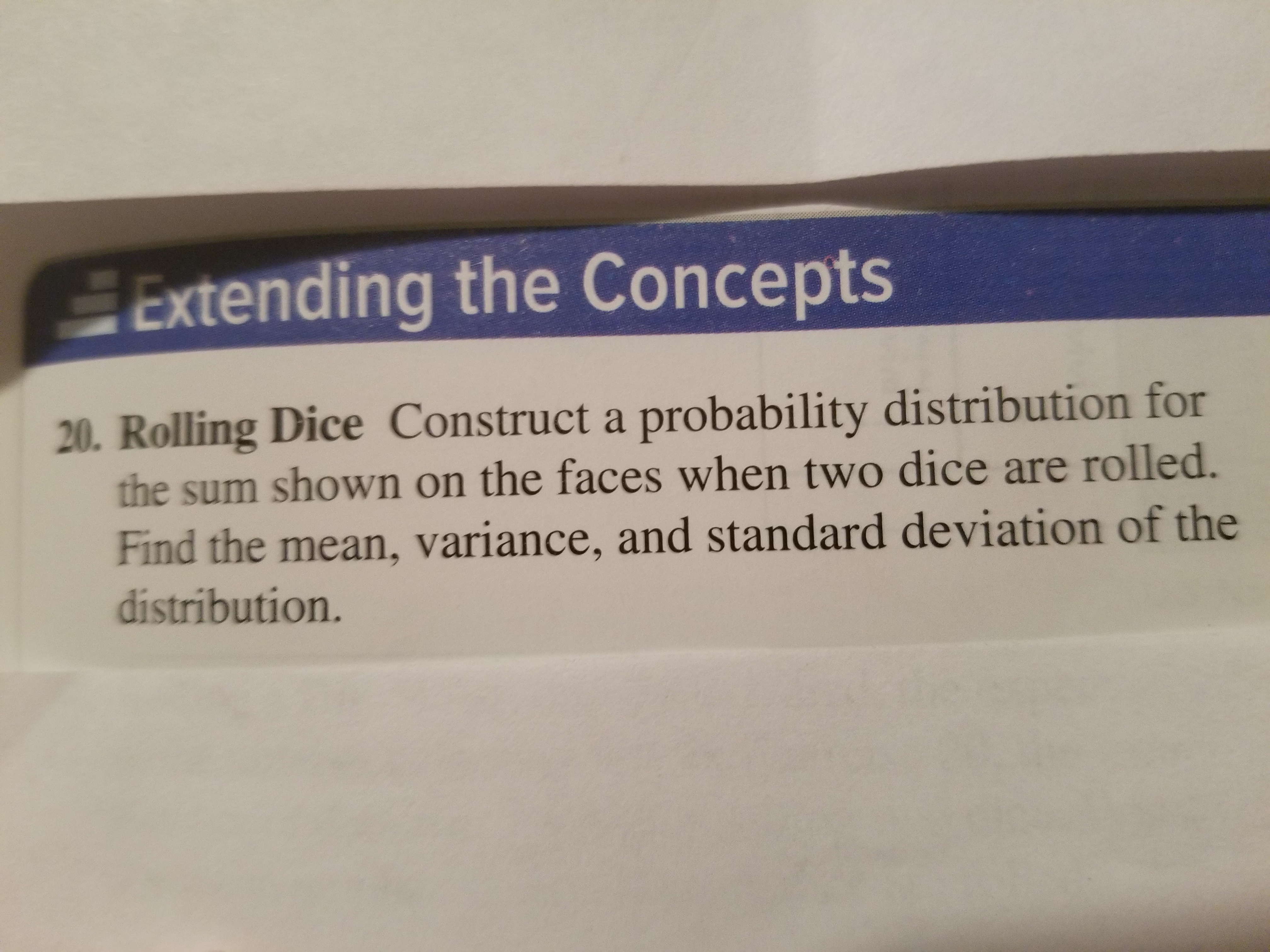# Extending the Concepts20. Rolling Dice Construct a probability distribution forthe sum shown on the faces when two dice are rolled.Find the mean, variance, and standard deviation of thedistribution.

Questionhelp_outlineImage TranscriptioncloseExtending the Concepts 20. Rolling Dice Construct a probability distribution for the sum shown on the faces when two dice are rolled. Find the mean, variance, and standard deviation of the distribution. fullscreen
check_circleExpert Solution
Step 1

Sample space:

The set of all possible outcomes of a probability experiment is called sample space of the experiment.

Here, two dice are rolled.

The total number of outcomes in the sample space be n(S) = 62 = 36.

Outcomes will be occurred in ordered pairs. First number in each ordered pair represents the number on the first die and second number in each ordered pair represents the number on the second die. Each of the two numbers can take values 1 to 6.

The sum of the numbers on the dice will be 2, 3, 4, 5, 6, 7, 8, 9, 10, 11 and 12.

The sample space for the experiment is given below:

Step 2

The general formula to obtain probability of an event A is,

P(A) = (number of favourable elements for event A)/(Total number of elements in the sample space).

Here, X be the sum shown on the face when two dice are rolled. The probability distribution is obtained by dividing the number of favourable cases with total number of elements in the sample space:

Step 3

The formula for the mean of a dis...

### Want to see the full answer?

See Solution

#### Want to see this answer and more?

Solutions are written by subject experts who are available 24/7. Questions are typically answered within 1 hour*

See Solution
*Response times may vary by subject and question
Tagged in

### Statistics